# Bivariate Data Analysis and Graphs

Travis Hartin, Cathryn Jackson
• Author
Travis Hartin

Travis has taught college-level statistics, research methods, and psychology courses for eight years. Travis has a Master’s degree and PhD in experimental psychology from Kent State University with a focus on student learning and cognitive research.

• Instructor
Cathryn Jackson

Cat has taught a variety of subjects, including communications, mathematics, and technology. Cat has a master's degree in education and is currently working on her Ph.D.

Learn what bivariate data is. See a comparison of univariate vs. bivariate. Understand what a bivariate analysis is. Updated: 12/13/2021

Show

## What is Bivariate Data?

In a scientific study, researchers collect information about their variables of interest in the form of data. The data that is collected can be univariate or bivariate, depending on the nature of the study.

• Univariate data: Uni means one and variate is another word for a variable. This refers to an instance in which a single variable is examined or described. For example, a researcher may measure the amount of time it takes people to complete a crossword puzzle as the sole variable of interest.
• Bivariate data: Bi means two. Therefore, bivariate data involves studying and comparing two separate variables. For example, a researcher may record how long it takes people to complete a crossword puzzle while measuring the stress levels of the participants. In this example, the two variables that the researcher is examining are time and stress.

### Univariate vs. Bivariate

As previously mentioned, univariate data involves collecting information about a single variable. Here are more examples of univariate data:

• Recording gender as students enter a room.
• Recording age as voters enter a polling booth.
• Asking participants to report their favorite type of music.

Univariate data is often used to understand the characteristics of a population, such as central tendency and variability. It can also be used to describe the basic findings of an experiment, such as reporting the mean and standard deviation for individual variables.

Univariate data analysis is not used to compare the relationship between different variables. To do so, researchers use bivariate data analyses.

## Bivariate Data

Mindy is a college student who works as a teacher's assistant at an elementary school. She is helping the third grade teacher grade a reading test. Mindy notices that the grades on the reading test are all over the place, meaning that there are some students who did very well, some students who did average and some students who did poorly. These are the results of the test: 55, 32, 67, 100, 98, 75, 46, 82, 72, 93, 44, 26, 67.

Later, Mindy is grading a questionnaire. The students are answering questions about what they do at home. One of the questions asks the student to track how much they read outside of school. These are the number of hours that each student reported on his or her questionnaire: 1, 2, 0, 3, 4, 6, 1, 2, 5, 0, 1, 1, 2.

Mindy wonders if there is a relationship between the number of hours a student spends each week reading and the reading test scores.

In this lesson, you will be learning about the definition and uses of bivariate data. We will also compare and contrast the characteristics of univariate data and bivariate data.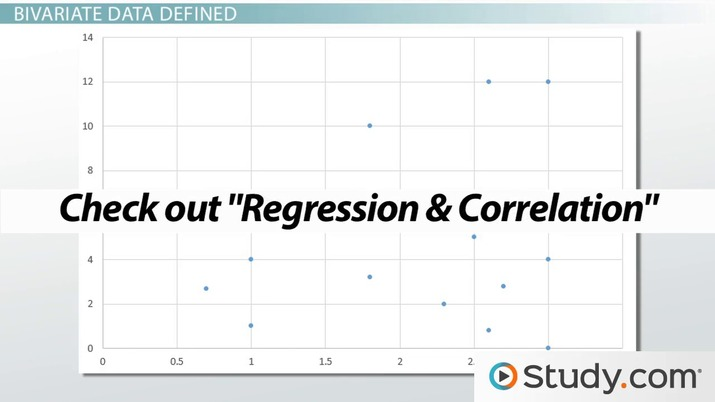An error occurred trying to load this video.

Try refreshing the page, or contact customer support.

Coming up next: What is a Two-Way Table?

### You're on a roll. Keep up the good work!

Replay
Your next lesson will play in 10 seconds
• 0:01 Bivariate Data
• 1:00 Bivariate Data Defined
• 4:07 Understanding…
• 6:15 Lesson Summary
Save Save

Want to watch this again later?

Timeline
Autoplay
Autoplay
Speed Speed

## Bivariate Relationship

Bivariate data is used to look for relationships between variables. One of the main purposes of determining whether or not two variables are related is to see if one variable causes the other. A causal link between two variables is typically found by determining if changes in one variable are caused by changes in another. This type of research involves two basic types of variables:

• Independent variable: The variable that a researcher manipulates in an experiment. The researcher predicts that changing the independent variable causes a meaningful change in another variable.
• Dependent variable: The variable that a researcher predicts will change by manipulating the independent variable. The important thing to note is that the researcher does not directly change this variable; it is changed or controlled by an outside factor (often the independent variable).

For example, a researcher is interested in whether or not students' attitudes toward a statistics class change based on the time of the class. To answer this question, the research measures students' attitudes from a morning class and an evening class. The researcher finds that students in the evening class have a more positive attitude toward statistics than students in the morning class.

What are the independent and dependent variables in the previous example? The independent variable is the time of the class because it is the variable that was manipulated by the researcher. Students' attitudes toward statistics are the dependent variable, or the variable that the researcher predicted might change in relation to the independent variable.

### Bivariate Data Examples

Now, take a moment to look at a few bivariate data examples.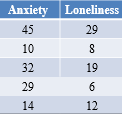Figure 1 shows the results of a study that measured anxiety levels and feelings of loneliness.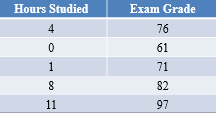Figure 2 shows the results of a study that compared the amount of time students spent studying and their exam grades.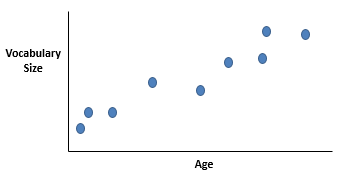Figure 3 is a graphical representation of bivariate data. It shows the relationship between age and vocabulary scores in a sample of preschoolers. We will return to the idea of graphing bivariate data later.

## What is a Bivariate Analysis?

Data analysis is the process of organizing, describing, and evaluating data in order to look for information that might help researchers make conclusions about various phenomena. It is used to help determine whether a particular research finding represents a real-world quality or some type of statistical fluke or error.

## Types of Analysis for Bivariate Data

There are different bivariate data analyses.

### Scatter Plot

A scatter plot is a graph used to show the values associated with two variables. As an example, take a moment to look at the graph from a previous example:In this particular example, we have already identified age as the independent variable and vocabulary as the dependent variable. Notice that the independent is on the horizontal axis (i.e., the x-axis) and the dependent variable is on the vertical axis (i.e., the y-axis). This is a rule-of-thumb you should keep in mind when making and interpreting these types of graphs.

Take a moment to examine the following example for how to create a scatter plot.

Imagine you are interested in whether or not running influences how much people sleep. To answer this question, you have people run as many laps as they can and then record how many hours they sleep the following night. You obtained the following data: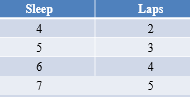Let's graph this data on a scatter plot one data point at a time. Each participant will be represented on the graph in accord to where X (i.e., laps ran) and Y (i.e., hours slept) intercept. First, plot the point where X=2 and Y=4 meet on the graph.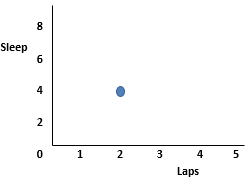Then, plot the point where X=3 and Y=5 meet on the graph.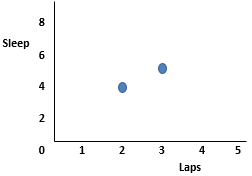Do the same for where X=4 and Y=6 meet on the graph.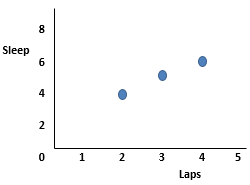Finally, plot the point where X=5 and Y=7 meet on the graph.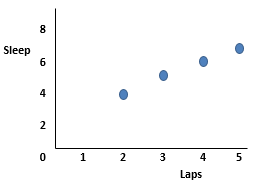## Bivariate Data Defined

Bivariate data deals with two variables that can change and are compared to find relationships. If one variable is influencing another variable, then you will have bivariate data that has an independent and a dependent variable. This is because one variable depends on the other for change. An independent variable is a condition or piece of data in an experiment that can be controlled or changed. A dependent variable is a condition or piece of data in an experiment that is controlled or influenced by an outside factor, most often the independent variable.

This is very different from univariate data, which is one variable in a data set that is analyzed to describe a scenario or experiment.

For example, if Mindy was studying for a college test and tracks her study time and her test scores, she might see that the more time she spends studying, the better her test scores become. Therefore, in this scenario, Mindy's test scores are the dependent variable because they depend on the number of hours she studies. Likewise, the number of study hours would be considered the independent variable. For that reason, we can see the relationship in this bivariate data set: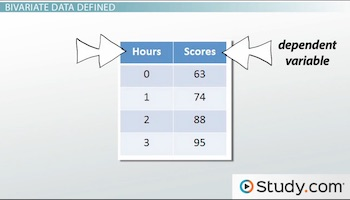In this case, we can compare the number of hours the third grade students spend reading with his or her test score, like this: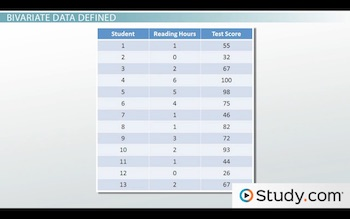We can also display this data visually, like this: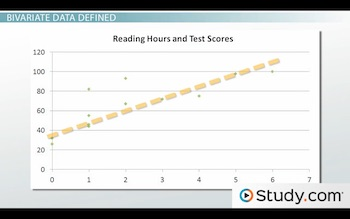Notice that most of the points increase both vertically and horizontally. You may notice that we have graphed the number of reading hours on the x-axis, horizontally, and the test scores on the y-axis, vertically. When a bivariate data set shows an overall increase in numbers like this, it is called a positive correlation, where the dependent variables and independent variables in a data set increase or decrease together.

This means that there is a positive relationship between the number of hours spent reading during the week and the test score of the student. In other words, the more a student reads, the better they score on the reading test. Therefore, in this case the independent variable is the amount the student reads during the week, because that is something they can control. The dependent variable is the score on the test; they can only control this variable if they change the independent variable.

If the numbers sloped downward, like the bivariate data in the graph below, then you have a data set with a negative correlation, where the dependent variables and independent variables in a data set either increase or decrease opposite from one another. That means if the independent variable decreases, then the dependent variable would increase and vice versa.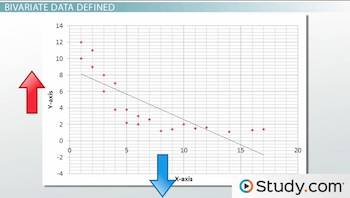If there is no relationship between the numbers, as shown in the graph below, then the data set has no correlation. You can learn more about correlation in the Regression & Correlation Chapter of this course!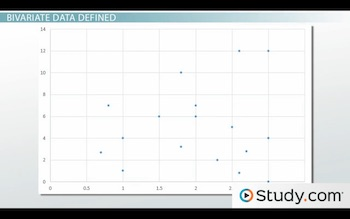## Understanding Bivariate and Univariate Data

The third graders in Mindy's class are studying plants. Each student records the amount of water they give the plant each day and the height of the plant. Are the students studying bivariate data? Yes, they are studying two separate variables in which each variable can change.

To unlock this lesson you must be a Study.com Member.

Video Transcript

## Bivariate Data

Mindy is a college student who works as a teacher's assistant at an elementary school. She is helping the third grade teacher grade a reading test. Mindy notices that the grades on the reading test are all over the place, meaning that there are some students who did very well, some students who did average and some students who did poorly. These are the results of the test: 55, 32, 67, 100, 98, 75, 46, 82, 72, 93, 44, 26, 67.

Later, Mindy is grading a questionnaire. The students are answering questions about what they do at home. One of the questions asks the student to track how much they read outside of school. These are the number of hours that each student reported on his or her questionnaire: 1, 2, 0, 3, 4, 6, 1, 2, 5, 0, 1, 1, 2.

Mindy wonders if there is a relationship between the number of hours a student spends each week reading and the reading test scores.

In this lesson, you will be learning about the definition and uses of bivariate data. We will also compare and contrast the characteristics of univariate data and bivariate data.

## Bivariate Data Defined

Bivariate data deals with two variables that can change and are compared to find relationships. If one variable is influencing another variable, then you will have bivariate data that has an independent and a dependent variable. This is because one variable depends on the other for change. An independent variable is a condition or piece of data in an experiment that can be controlled or changed. A dependent variable is a condition or piece of data in an experiment that is controlled or influenced by an outside factor, most often the independent variable.

This is very different from univariate data, which is one variable in a data set that is analyzed to describe a scenario or experiment.

For example, if Mindy was studying for a college test and tracks her study time and her test scores, she might see that the more time she spends studying, the better her test scores become. Therefore, in this scenario, Mindy's test scores are the dependent variable because they depend on the number of hours she studies. Likewise, the number of study hours would be considered the independent variable. For that reason, we can see the relationship in this bivariate data set:In this case, we can compare the number of hours the third grade students spend reading with his or her test score, like this:We can also display this data visually, like this:Notice that most of the points increase both vertically and horizontally. You may notice that we have graphed the number of reading hours on the x-axis, horizontally, and the test scores on the y-axis, vertically. When a bivariate data set shows an overall increase in numbers like this, it is called a positive correlation, where the dependent variables and independent variables in a data set increase or decrease together.

This means that there is a positive relationship between the number of hours spent reading during the week and the test score of the student. In other words, the more a student reads, the better they score on the reading test. Therefore, in this case the independent variable is the amount the student reads during the week, because that is something they can control. The dependent variable is the score on the test; they can only control this variable if they change the independent variable.

If the numbers sloped downward, like the bivariate data in the graph below, then you have a data set with a negative correlation, where the dependent variables and independent variables in a data set either increase or decrease opposite from one another. That means if the independent variable decreases, then the dependent variable would increase and vice versa.If there is no relationship between the numbers, as shown in the graph below, then the data set has no correlation. You can learn more about correlation in the Regression & Correlation Chapter of this course!## Understanding Bivariate and Univariate Data

The third graders in Mindy's class are studying plants. Each student records the amount of water they give the plant each day and the height of the plant. Are the students studying bivariate data? Yes, they are studying two separate variables in which each variable can change.

To unlock this lesson you must be a Study.com Member.

#### What does bivariate mean in statistics?

"Bi" means two and "variate" is another word for a variable. So, bivariate refers to a statistical analysis that involves the comparison of two separate variables.

#### What is bivariate data analysis?

Bivariate data analysis is a statistical test that involves two separate variables. It is used to determine whether or not two variables are related.

#### What are the uses of bivariate data?

Bivariate data can be used to determine whether or not two variables are related. If a relationship between variables is established, bivariate data is used to determine if there is a causal link between those variables.

#### What is an example of bivariate data?

An example of bivariate data is data collected from a study that compares levels of anxiety to the number of times participants pace back and forth in a room.

### Register to view this lesson

Are you a student or a teacher?